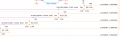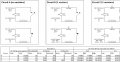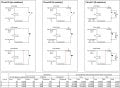# Lowering voltage on the Vout produced by voltage divider

Joined Nov 29, 2018
40
Hi folks,

I have what I think is a basic question but I've not been able to find an answer on my own and haven't been able to come up with the answer otherwise on my breadboard, I'm also a network/systems/software/database engineer type but not a hardware or electrical engineer though I'm learning.

I have a series of 12V .4mA circuits that I want to connect both to the GPIO on a Raspberry Pi and to an ADC, instead of having the RPI constantly polling the ADC I'll use the GPIO transitions to/from "low" and "high" as a method to replicate normal interrupt behavior. The RPI GPIO are 0 - 3.3V with 0 - 0.7V being the range for "low" and 2.4 - 3.3V being the range for "high" while the ADC channels are 0 - 2.5V.

Each of the circuits in the diagram are the same, we can disregard the purple lines with resistors. Using the bottom most circuit as an example, the one showing R11, R12 & R13. R11 & R12 are being used as a voltage divider to sufficiently and proportionally reduce the incoming circuit from 12V to something less than 3.3V; by my calculations with these at 1% tolerance Vout here should be 3.268V. This Vout is routed to the corresponding RPI GPIO which is the portion of the circuit exiting the diagram to the left. This Vout is also "tapped" by the circuit where R13 sits and this tapped run terminates to the corresponding ADC channel.

The issue I am struggling with is how to proportionally reduce the voltage at this point though I suspect I need another voltage divider to pull the 0-3.3V value into the 0 - 2.5V range. Secondarily I'm wondering if there is a better or more appropriate method for doing this given that eventually I'll have this built into a PCB.

Appreciate the feedback and guidance.#### Yaakov

Joined Jan 27, 2019
1,611
If you set your divider to max out at 3.2, a signal diode on the ADC input will drop it .7 volts more, to 2.5.

Joined Nov 29, 2018
40
If you set your divider to max out at 3.2, a signal diode on the ADC input will drop it .7 volts more, to 2.5.
Thanks for the quick response Yaakov, I'll research but if I understand you correctly a signal diode would reduce the voltage by a fixed amount. A .8v signal diode would get me from 3.25V to 2.45V. is that a better way to also reduce from the 12V to 3.25V?

#### Yaakov

Joined Jan 27, 2019
1,611
Thanks for the quick response Yaakov, I'll research but if I understand you correctly a signal diode would reduce the voltage by a fixed amount. A .8v signal diode would get me from 3.25V to 2.45V. is that a better way to also reduce from the 12V to 3.25V?
The diodes junction drops the voltage by a fixed amount. A silicon junction drops .7V (that’s no coincidence concerning the low value for the DIO). Since the high has a substantial range, I would be inclined to scale it so that you have a full scale voltage to the ADC and a solid high for the DIO. You might want a 10-turn trimmer on the ADC to set the scale as well.

This is just how I might approach it, I await other answers to you, someone might have a much better one. Also, you probably need pull down resistors on the DIO lines to ensure they hold solid logic levels when low.

#### ericgibbs

Joined Jan 29, 2010
9,116
hi sp.
If I am reading your intro notes correctly, R13 is connecting to the off circuit diagram of an ADC input, so it is in series with the 3.3V pd source.???
What is the input impedance of the ADC input pin.??
E

#### Yaakov

Joined Jan 27, 2019
1,611
Thinking about it, I would expect that feeding the DIOs with the output of comparators and focusing on signal conditioning specifically for the ADC is probably a better route than adapting the output to both by kludging it.

•#### AnalogKid

Joined Aug 1, 2013
8,228
To make your question more clear, please post a sketch of just the circuit in question. Inputs from the left, outputs to the right, and all grounds point down.

ak

#### crutschow

Joined Mar 14, 2008
23,800
The diodes junction drops the voltage by a fixed amount. A silicon junction drops .7V
That's a common assertion but I wouldn't call the log relation between a diode's voltage and current as giving a fixed voltage.
Actually, at the typical small (mA or so) currents of silicon signal diodes in a circuit, the voltage drop is around 0.6V, but it does vary with current and is not useful if you do indeed need a fixed voltage drop for measuring an analog voltage to any significant accuracy (within less than a tenth of a volt or so).
At the larger currents that larger rectifier diodes operate at, the forward drop is higher of course (0.7V at around 50mA, for example).

•shortbus and Yaakov

#### BobTPH

Joined Jun 5, 2013
2,137
At the very low current drawn by a GPIO input. The diode drop will not be 0.6V.

Bob

Joined Nov 29, 2018
40
Thinking about it, I would expect that feeding the DIOs with the output of comparators and focusing on signal conditioning specifically for the ADC is probably a better route than adapting the output to both by kludging it.
Hi Yaakov - I didn't understand your response Tuesday though I think now I understand it, at least partially if not fully. I also think I may have been trying to solution this differently than I perhaps should have.

What I am trying to do is monitor the status of several circuits individually to determine if the circuit is open, closed or shorted. When I started a few weeks ago I was thinking I'd be able to use voltage to do this by sending 12VDC/.02A out each circuit and measuring what is coming back, something that I have learned would be monitoring on the "low side". The challenge is the circuits are already installed and some of them have no resistor, some have 1 resistor and others have 2. The diagram I've attached represents a simple version of each circuit scenario.

Your comment led to more research which led to, I think, an aha moment. It forced me to go back to my calculations and the testing i've been doing with my bench power supply, MM and so on in an attempt to validate things. I realized that I didn't recognize high/low side and I wasn't correctly calculating the voltage i'd see given different resistances. I believer in the diagram I've correctly applied ohms law. If I have correctly applied it then that presents some challenges for me and brings me back to your note about comparators which led me to op-amps, both of which I am still researching & learning about.

Regarding the attached diagram:

Circuit A - not much of an issue; either V & A show up on the low side when the circuit (switch) is closed or they don't when the circuit (switch) is open. I do question what I would see if I actually monitored V & A on the high side both in open & closed switch states?

Circuit B - with the single 5600 ohm 20% resistor added I would see between 90V and 134V and between .0018A and .0027A when the circuit (switch) is closed and I am monitoring at the low side. Not clear what I'd see if I actually monitored for the same on the high side.

Circuit C - with two 5600 ohm 20% resistors. When the circuit (switch) is closed I would see the same numbers on the low side as I do for the low side of Circuit B, 90V-134V and .0018A-.0027A; R2 isn't involved, voltage & current flow through the switch instead of R2. When the circuit (switch) is open I would see between 179.6V-269.2V and between .0009A-.0013A as both R1 & R2 are involved.

If my calculations are correct, which is a question I am asking here, then I really should be looking to measure current instead of voltage on the low side because the voltage I'll see will be between 0V - 269.2V whereas the current I'll see will be between 0A - 0.6A. If I needed to use the measured value to control the high/low status of a GPIO pin I could more easily and accurately (i presume) scale 0A-.6A to the 2.4V-3.3V range I would need to hold the pin high or the 0V-.7V range I would need to hold the pin low. At the same time the output from the comparator or op amp could be input to a channel on the ADC giving me the ability to read it (the GPIO pin high/low state change would tell me I needed to read it) giving me the ability to use the value in my programming.

My questions are;

1. Am I correctly calculating things based on ohms law?
2. How would I go about calculating what I should expect to see on the high side?
3. Have I correctly understood your point with respect to comparators?

thx - sean#### Yaakov

Joined Jan 27, 2019
1,611
My questions are;

1. Am I correctly calculating things based on ohms law?
2. How would I go about calculating what I should expect to see on the high side?
3. Have I correctly understood your point with respect to comparators?

thx - sean
Hello. I've only been able to scan this briefly as I am running around today and won't have time to get back to it until, probably, Sunday but from what I saw, and I might be wrong, I want to clarify the comparator comment.

The general idea is the comparator would watch the analog signal of interest and according to the scaling you choose, it would produce a definite 0 or 1 output scaled to your DIOs low and high. That is, if the comparator sees anything less than what you consider ON, the output would be pulled low, effectively 0V while if it sees at least whatever you consider ON, it's output would be a solid high, say 5V or whatever.

There would be no analog vagaries from the point of view of the DIO.

This frees you to provide, independently, whatever is best for the ADC, ignoring the DIO requirements.

I hope that fits with your thinking, I will review this when I get the chance.

Joined Nov 29, 2018
40
Ah! No rush, appreciate the consideration. In the meantime I will look into the DIO.

#### eetech00

Joined Jun 8, 2013
1,740
Hi folks,

I have what I think is a basic question but I've not been able to find an answer on my own and haven't been able to come up with the answer otherwise on my breadboard, I'm also a network/systems/software/database engineer type but not a hardware or electrical engineer though I'm learning.

I have a series of 12V .4mA circuits that I want to connect both to the GPIO on a Raspberry Pi and to an ADC, instead of having the RPI constantly polling the ADC I'll use the GPIO transitions to/from "low" and "high" as a method to replicate normal interrupt behavior. The RPI GPIO are 0 - 3.3V with 0 - 0.7V being the range for "low" and 2.4 - 3.3V being the range for "high" while the ADC channels are 0 - 2.5V.

Each of the circuits in the diagram are the same, we can disregard the purple lines with resistors. Using the bottom most circuit as an example, the one showing R11, R12 & R13. R11 & R12 are being used as a voltage divider to sufficiently and proportionally reduce the incoming circuit from 12V to something less than 3.3V; by my calculations with these at 1% tolerance Vout here should be 3.268V. This Vout is routed to the corresponding RPI GPIO which is the portion of the circuit exiting the diagram to the left. This Vout is also "tapped" by the circuit where R13 sits and this tapped run terminates to the corresponding ADC channel.

The issue I am struggling with is how to proportionally reduce the voltage at this point though I suspect I need another voltage divider to pull the 0-3.3V value into the 0 - 2.5V range. Secondarily I'm wondering if there is a better or more appropriate method for doing this given that eventually I'll have this built into a PCB.

Appreciate the feedback and guidance.

View attachment 171600
Hi

That is, is your ADC looking for a change of state represented by two voltage levels (digital)? or does it need to read a voltage range?

eT

Joined Nov 29, 2018
40
Hi

That is, is your ADC looking for a change of state represented by two voltage levels (digital)? or does it need to read a voltage range?

eT

I noticed I had an error in the prior attachment as I wasn't showing the correct V/A values for Circuit C when the reed switch is open. I also decided to add diagrams showing each circuit when the reed switch itself has been bypassed though I labeled that as being shorted. The potential for someone to bypass the reed switch is the reason for the resistor at the reed switch in Circuit C.#### eetech00

Joined Jun 8, 2013
1,740

I noticed I had an error in the prior attachment as I wasn't showing the correct V/A values for Circuit C when the reed switch is open. I also decided to add diagrams showing each circuit when the reed switch itself has been bypassed though I labeled that as being shorted. The potential for someone to bypass the reed switch is the reason for the resistor at the reed switch in Circuit C.

View attachment 171874
Perhaps you can install a current sense resistor of a small known value, then read the voltage drop across it with the ADC. The ADC would expect specific values for open, short, or "OK".

Just a thought...

eT

Joined Nov 29, 2018
40
Perhaps you can install a current sense resistor of a small known value, then read the voltage drop across it with the ADC. The ADC would expect specific values for open, short, or "OK".

Just a thought...

eT
I appreciate the input, am currently reading about sense resistors az well as an EE article about high side and low side current sensing.

Joined Nov 29, 2018
40
just an FYI - I think i've determined that I am not properly calculating the voltage drop across each resistor which i'll work on later today and post with a request to validate. Just didn't want someone looking at the question and answering based on my faulty #s above.

Joined Nov 29, 2018
40
Believe I've worked out the voltage drop across the resistors correctly but if someone could confirm that for me I would greatly appreciate it.

1. With the circuit being 12V & .02A (ie: 20 mA) each 5600 ohm resistor will reduce the voltage by 112V (5600 * .02 = 112); to be technically accurate if it is a resistor with 20% tolerance then the voltage reduced would be between 89.6V and 134.4V.
2. If #1 is correct does voltage actually go negative? When Circuit B is closed is the voltage between R1 and - actually going to be 112V - 12V so... -100V?
Once I've been straightened out on this basic circuit mathematics I'll move back to my original intent which is measuring voltage and/or current on each circuit and determining the best way to accomplish that.

I greatly appreciate the feedback...and patiencethx - sean